Next: Equivalence of the order Up: 1: WHAT IS A Previous: Functions with discontinuities

## Fourier transformations

Very simple examples of Fourier transformations for functions with discontinuities are given Table 2.
 2|c|Table 2: Fourier Transformations e-| t |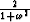e-t.H(t)H(t)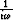t e-t.H(t)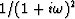It is very simple to determine the Fourier transforms of all the functions in Table 1 using these simple properties of the Fourier transformation: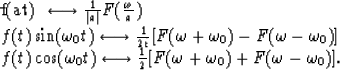We can see from the Table 2 that the Fourier transforms of functions with discontinuities of order 0 behave atas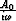.Meanwhile, in case of a discontinuity of order 1,behaves asymptotically as. Let us take, for instance, the third function from Table 1: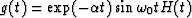. In this case,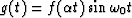where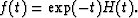Thenand whenMore generally, it is simple to prove that, if(i.e., is continuous), and if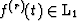(i.e., is summable), then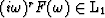.Consequently, when,,this convergence being not slower than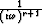.

We shall prove the following statement. Let f(t) be a right-sided function, and, let's suppose that, for all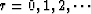,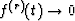(when) in the same way as exp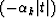. Then:(2)

As f(t) is a right-sided function, we have:(3)
Let us integrate this integral by parts: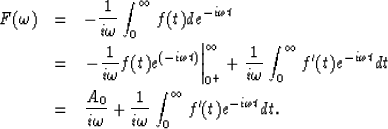(4) (5) (6)

Repeating this N times we obtain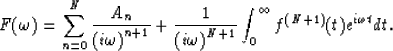(7)

By definition of the order of discontinuity. From the condition concerning the behavior of f(k)(t) at, the integral on the righthand side of equation (7) converges to at. This means that the last equation is equivalent to equation (2). So the asymptotic behavior of the Fourier-transforms for high frequencies reflects the sharpness of the discontinuities of the (original) functions. Both notions (asymptotic behavior and discontinuities) are equivalent ones.Next: Equivalence of the order Up: 1: WHAT IS A Previous: Functions with discontinuities
Stanford Exploration Project
1/13/1998